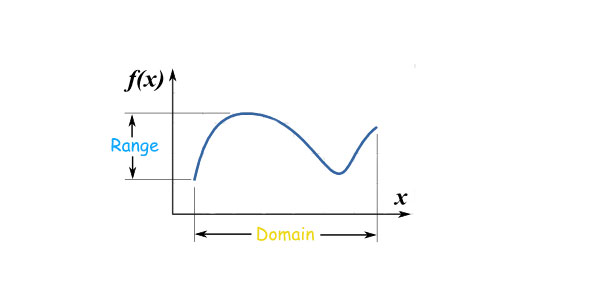# Unit 2 Review - Domain And Range

6 Questions | Total Attempts: 57SettingsIn mathematics, the domain of a function is defined as the complete set of possible values for an independent variable. The range is the difference between the highest and lowest of those possible values. What else can you tell us?

• 1.
Review #1: State the domain and range.
• A.

D: {-3, -2, 0, 1, 3} R: {-6, 4, 5, 6, 10}

• B.

D: {-6, 4, 5, 6, 10} R: {-3, -2, 0, 1, 3}

• C.

D: {-6, -3, -2, 0, 1, 3, 4, 5, 6, 10} R: {-6, -3, -2, 0, 1, 3, 4, 5, 6, 10}

• D.

{-3, 6, 0, 4, 3} R: {5, -2, -6, 1, 10}

• 2.
Review #2: Find the range of the equation.
• A.

{-2, 1, 0}

• B.

{-0.5, 1, 2}

• C.

{-4, 2, 3}

• D.

{-2, -1, 0.5}

• 3.
Review #3: what is the range of the function shown on the graph?
• A.

-6 less than x less than 0

• B.

-6 less than y less than 0

• C.

-4 less than x less than 8

• D.

-4 less than y less than 8

• 4.
Review #4: What is the domain of the function shown in the graph?
• A.

-6 (less than or equal) y (less than) 5

• B.

-6 (less than or equal) x (less than) 5

• C.

-4 (less than or equal) y (less than) 0

• D.

-4 (less than or equal) x (less than) 0

• 5.
Review #5: State the domain and range of the graph shown below.
• A.

D: all reals R: y (greater than or equal) 4

• B.

D: all reals R: y (less than or equal) 4

• C.

D: x (less than or equal) 4 R: all reals

• D.

D: x (greater than or equal) 4 R: all reals

• 6.
Review #6: State the domain and range.
• A.

D: {-5, 0, 1, 2, 8} R: {-4, -2, 0, 2, 6}

• B.

D: {0, 2, 4, 6} R: {0, 1, 2, 5, 8}

• C.

D: {-4, -2, 0, 2, 6} R: {-5, 0, 1, 2, 8}

• D.

D: {-4, 0, 1, 2, 6} R: {-5, -2, 0, 2, 8}

Related TopicsBack to top